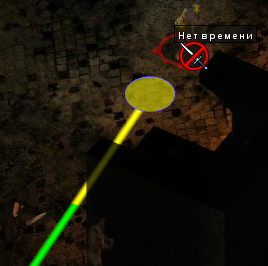# How to go to the neighboring node? Is there an easy way to colorize a Line Renderer?

Hello everyone.

I’m trying to make the character stop at a certain distance from the object. Here is my code.

``````if (hit.collider.gameObject.CompareTag("Versys") | hit.collider.gameObject.CompareTag( "Unit"))
{
Vector3 cellTochka = gameObject.transform.position - hit.collider.gameObject.transform.position;
var distance = cellTochka.magnitude;
var direction = cellTochka / distance;
var vv = direction * (distance - rasstoyanieYdara);
var tochkaYdara2 = gameObject.transform.position - vv;
nodeM2S = AstarPath.active.GetNearest(tochkaYdara2, NNConstraint.Default).node;
Vector3 tochkaYdaraProm = (Vector3)nodeM2S.position;
float rasstTy = Vector3.Distance(tochkaYdaraProm, hit.collider.transform.position);
if (rasstTy <= rasstoyanieYdara + zazorydara) tochkaYdara = tochkaYdaraProm;
else
{
??????????
}
}
``````

It is necessary that the character gets into the circle. What can be used to select the neighboring node at the end of the path? Or is my way wrong? Is the size of the knot suitable for a character of this size?

And the second question.Is there an easy way to colorize a line depending on the length of the path? Like on a picture. Here is the code how it is rendered.

``````AILerp ai2;
LineRenderer lin;

ai2.GetRemainingPath(buffer, out bool stale);
lin.positionCount = buffer.Count;
lin.SetPositions(buffer.ToArray());
``````

I solved the first problem. Initially, my approach was wrong. It takes a lot of time without prompts. During a small test, everything works well.
I did:

1. Two colliders. One for hovering the mouse, the other for forming obstacles.
2. When hovering the mouse over an object, removed obstacles from it.
3. Calculate the path from the target to the object.
4. Found the nearest point on the way from the target to the object that exceeds the required distance. Also calculated the distance from the point to the target.
5. Found a direction vector from this point to the target.
6. Found the distance that you need to go along this vector. And calculated the coordinates of the desired node.
7. I checked if this node is in the circle.
8. If the node is not in the circle. Then, through the BFS function, I found the nearest nodes and calculated the necessary node.
``````if (mysh == false && hit.collider.gameObject.CompareTag("Versys") | hit.collider.gameObject.CompareTag("Unit"))
{

GameObject navelsya = hit.collider.gameObject;
vragPoz = AstarPath.active.GetNearest(navelsya.transform.position, NNConstraint.Default).node;
navelsya.GetComponent<AILerp>().canMove = false;
if (!Input.GetMouseButtonDown(0) && mysh == false)
{
pytVragYa = navelsya.GetComponent<Seeker>().StartPath(navelsya.transform.position, gameObject.transform.position);
pytVragYa.BlockUntilCalculated();
}
navelsya.GetComponent<AILerp>().GetRemainingPath(buffer2, out bool stale);

Vector3 exper = new Vector3();
Vector3 experPer = new Vector3();
float D1D = 0;
float promD1D = 0;

if (navelsya.GetComponent<AILerp>().remainingDistance > rasstoyanieYdara)
{
for (int i = 0; i < buffer2.Count - 1; i++)
{
float D1 = Vector3.Distance(buffer2[i], buffer2[i + 1]);
D1D = D1D + D1;
if (D1D >= rasstoyanieYdara)
{
promD1D = D1D - rasstoyanieYdara;
experPer = buffer2[i];
exper = buffer2[i + 1];
break;
}

}

Vector3 cellTochkaQ = exper - experPer;
var distanceQ = cellTochkaQ.magnitude;
var directionQ = cellTochkaQ / distanceQ;
var vvQ = exper - directionQ * promD1D;
Vector3 tchk2 = new Vector3();
if (exper != Vector3.zero) // I don't know where it comes from, probably because of the cycle. This is to suppress the error.
{
nodeM2S = AstarPath.active.GetNearest(vvQ, NNConstraint.Default).node;
tchk2 = (Vector3)nodeM2S.position;
}
else
{
nodeM2S = AstarPath.active.GetNearest(navelsya.transform.position, NNConstraint.Default).node;
tchk2 = (Vector3)nodeM2S.position;
}

Vector3 tochkaYdaraProm = tchk2;
float rasstTy = Vector3.Distance(tochkaYdaraProm, hit.collider.transform.position);
if (rasstTy <= rasstoyanieYdara + zazorydara) tochkaYdara = tochkaYdaraProm;
else
{
for (int i = 0; i < radZazora.Count; i++)
{
if (rasdis <= rasstoyanieYdara)
{
break;
}
}

}

}
else
{
tochkaYdara = gameObject.transform.position; // Another piece of code is responsible for this option. This is just in case.
}
}
``````

During the tests, the objects behave as planned.
But the second question is still open. How to color a line? Use the way I wrote above? Or is there an easier option?

Hi

Coloring a line renderer is not something related to this package. I think you will have better luck posting in the Unity forums. I think you will have to add points right at the border between the green and yellow, and change all points before it to green, and all points after it to yellow.

1 Like

Thank you. There probably isn’t an easy way.